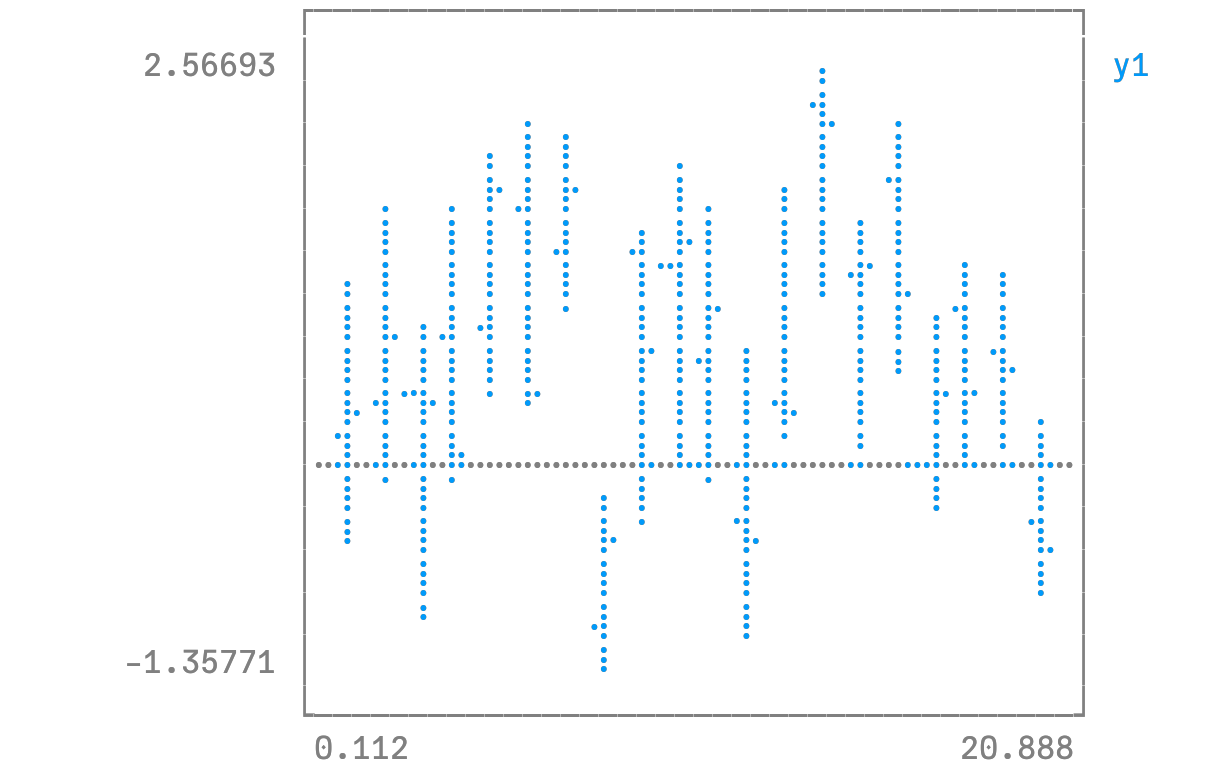# Open/High/Low/Closeusing Plots
unicodeplots()

Create an OHLC chart. Pass in a list of (open,high,low,close) tuples as your y argument. This uses recipes to first convert the tuples to OHLC objects, and subsequently create a :path series with the appropriate line segments.

n = 20
hgt = rand(n) .+ 1
bot = randn(n)
openpct = rand(n)
closepct = rand(n)
y = OHLC[(openpct[i] * hgt[i] + bot[i], bot[i] + hgt[i], bot[i], closepct[i] * hgt[i] + bot[i]) for i = 1:n]
ohlc(y)